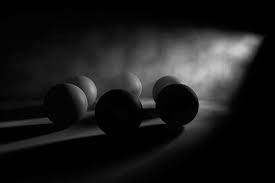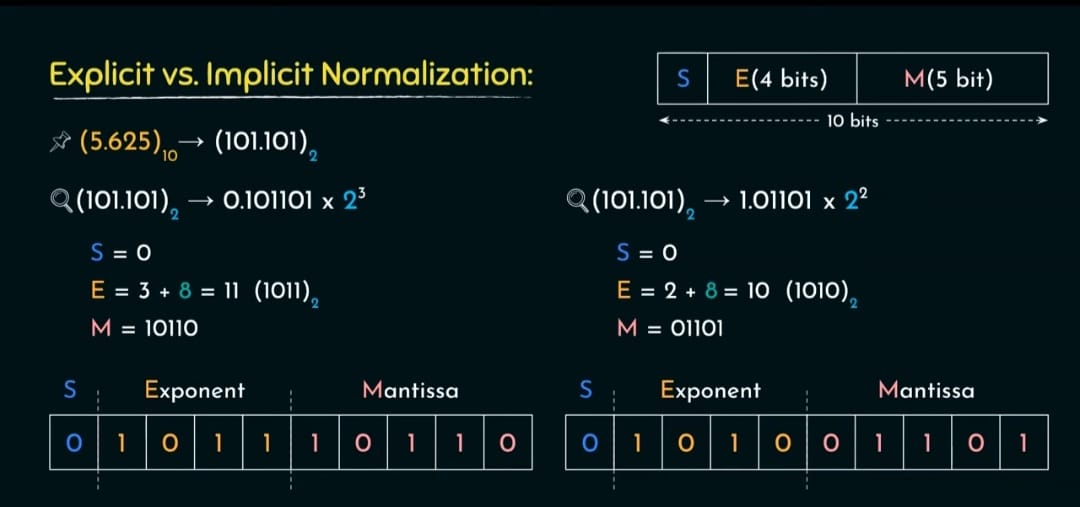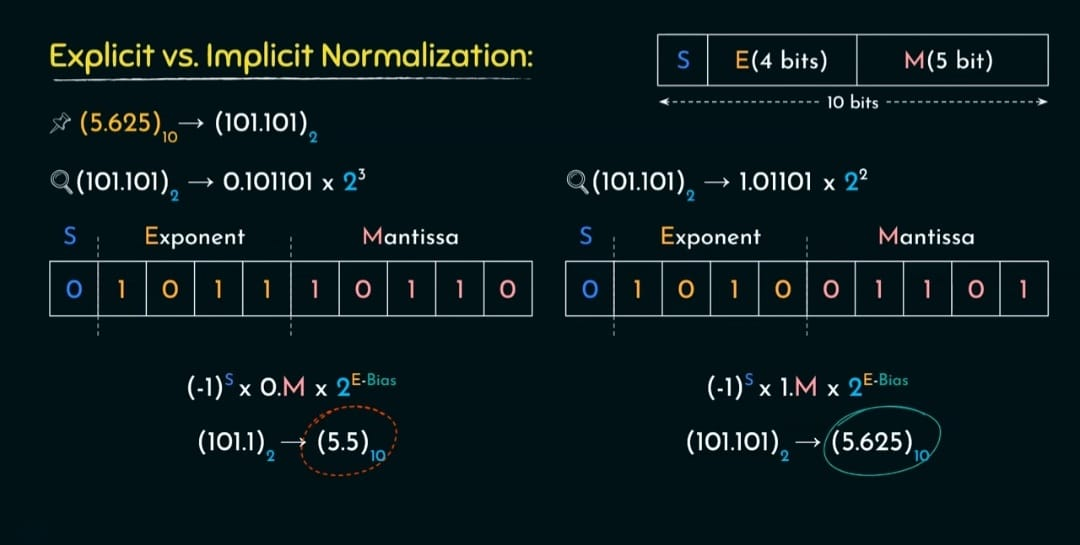# FLOATING POINT NUMBERS

There are two major approaches to store real numbers (i.e., numbers with fractional component) in modern computing. These are

(i) Fixed Point Notation

(ii) Floating Point Notation.

## Floating-Point Representation −

This representation does not reserve a specific number of bits for the integer part or the fractional part.Single-Precision floating-point number occupies 32-bits, so there is a compromise between the size of the mantissa and the size of the exponent.

### Applications of Floating Point Numbers

1. Gaming: Real-time games require high precision and accuracy in the simulation of physics and other complex systems. Floating point numbers are used to calculate the movements and interactions of game objects, as well as to create realistic animations and special effects.1. Machine learning: Machine learning algorithms often require large amounts of data and complex calculations. Floating point numbers are used to ensure precise and accurate computations in applications such as deep learning, natural language processing, and image recognition.1. Computer graphics: Computer graphics require high precision and accuracy to create realistic and visually appealing images. Floating point numbers are used to calculate the position, orientation, and lighting of objects in 3D space.## Overflow

The exponent is too large to be represented in the Exponent field

## underflow

The number is too small to be represented in the Exponent fieldTo reduce the chances of underflow/overflow, can use 64-bit Double-Precision arithmetic.

## To convert the floating point into decimal

we have 3 elements in a 32-bit floating point representation:

``````i) Sign

ii) Exponent

iii) Mantissa
``````

## IEEE Floating point Number Representation −

IEEE (Institute of Electrical and Electronics Engineers) has standardized Floating-Point Representation

So, actual number is (-1)^s*(1.m)*2(e-Bias) (implicit), where s is the sign bit, m is the mantissa, e is the exponent value, and Bias is the bias number.

According to IEEE 754 standard, the floating-point number is represented in following ways:

Half Precision (16 bit): 1 sign bit, 5 bit exponent, and 10 bit mantissa

Single Precision (32 bit): 1 sign bit, 8 bit exponent, and 23 bit mantissa

Double Precision (64 bit): 1 sign bit, 11 bit exponent, and 52 bit mantissa

Quadruple Precision (128 bit): 1 sign bit, 15 bit exponent, and 112 bit mantissa.

## Biasing

``````For n Bit Number, range of signed numbers :

-2^(n-1) to 2^(n-1)-1
``````

### Normalization

Two Types:

1)Explicit Normalization:

Move the radix point to the LHS of the most significant '1' in the bit sequence.

It should be in the form of 0.X

``````                 (101.101)subscript2 = 0.101101 x 2^3
For example let us consider that we want to represent the above number in 32 bits.
Since it is 32 bits, there will be 1 sign bit, 8 bit exponent, and 23 bit mantissa
Range of signed numbers if you have n bits: (-2^(n-1) to 2^(n-1)-1)
here Exponent should be represented by 8 bits so using 8 bits we can represent from -128 to 127 .
For normalizing we have to add 128.
(101.101)subscript2 = 0.101101 x 2^3
since the number is positive sign bit will be 0.
Exponent will be 3+128=131 in binary it will be 10000011
Mantisa will be 101101
``````

(-1)^s*(0.M)*2^(E-B)

Sign bit is 0

M is mantissa which is 101101

Exponent is 131

Bias is 128

(-1)^0*(0.101101)*2^(131-128) = 0.101101 x 2^3

2)Implicit Normalization: Move the radix point to the RHS of the most significant '1' in the bit sequence.

It should be in the form of 1.X

``````               Consider the example  (101.101)subscript2 = 1.01101 x 2^2
For example let us consider that we want to represent the above number in 32 bits.
Since it is 32 bits, there will be 1 sign bit, 8 bit exponent, and 23 bit mantissa
Range of signed numbers if you have n bits: (-2^(n-1) to 2^(n-1)-1)
here Exponent should be represented by 8 bits so using 8 bits we can represent from -128 to 127 .
For normalizing we have to add 128.
(101.101)subscript2 = 1.01101 x 2^2
since the number is positive sign bit will be 0.
Exponent will be 2+128=130 in binary it will be 10000010
Mantisa will be 01101
``````

(-1)^s*(1.M)*2^(E-B)

Sign bit is 0

M is mantissa which is 01101

Exponent is 130

Bias is 128

(-1)^0*(1.01101)*2^(130-128) = 0.101101 x 2^2

## Example : Convert -1.75*10^15 to IEEE standard (32 bits)

``````             First we will convert 10^15
2^x=10^15
Taking log on both sides
log(2^x)=log(10^15)
xlog2=15log10                   log2=0.3 and log10=1
0.3x=15
x=15*10/0.3*10
x=50
so 2^50=10^15

-(1.75)*10^15 in decimal = -(1.11)*2^50 in binary

Since the number is negative Sign bit will be 1. (1 bit)
Exponent is 50+128=178 , in binary it will be 10110010 (8 bit)
Mantisa =1100000000.....00 (23 bit)
``````

### Explicit Vs Implicit Normalization

For the same above exampleImplicit normalization is more precise than the Explicit normalization.

## What is Floating Point Unit(FPU)?

A floating-point unit (FPU, colloquially a math coprocessor) is a part of a computer system

specially designed to carry out operations on floating-point numbers.

Typical operations are addition, subtraction, multiplication, division, and square root.

## Why do we need floating point unit?

Image result for what is floating point unit in digital electronics

Floating point numbers are used to represent noninteger fractional numbers and are used in most engineering and technical calculations,

for example, 3.256, 2.1, and 0.0036. The most commonly used floating point standard is the IEEE standard.

## What is Floating Point Unit(FPU)?

A floating-point unit (FPU, colloquially a math coprocessor) is a part of a computer system

specially designed to carry out operations on floating-point numbers.

Typical operations are addition, subtraction, multiplication, division, and square root.

## Why do we need floating point unit?

Image result for what is floating point unit in digital electronics

Floating point numbers are used to represent noninteger fractional numbers and are used in most engineering and technical calculations,

for example, 3.256, 2.1, and 0.0036. The most commonly used floating point standard is the IEEE standard.